# Rediscussing on the Force-Power equivalence

in English and Chinese

Keying  Guan

Department of Mathematics, School of Science, Beijing Jiaotong University

Email: keying.guan@gmail.com

Abstract: This paper will further accurately describe the equivalence relationship between force and power, point out that Einstein gave the basic principle for calculating radiation pressure based on the relationship between force and power, and point out that due to the influence of the Maxwell-Bartoli radiation pressure formula, Arthur S. Eddington underestimated the important role of radiation pressure in the interior of stars.

Contents: I. Regarding whether force and power are vectors or scalars

II. Electromagnetic radiation intensity and light pressure, Einstein's important contribution to the                                                    theory of light pressure

III. Why can't the word power be found in Landau and Lifshitz's great tome of physics?

IV. Some inner understandings of human beings on "Power" and "Force"

V.  Summary

In the previous blog posts

2) Force is equivalent to Power 2022-07-02

the author reveals the "equivalent relationship between force and power" that is not recognized by the academic circles, although it is contained in analytical mechanics. Due to the deep-seated problems involved in physics and mechanics, it is necessary to repeatedly think and demonstrate. The author finds that there is still room for improvement and further clarification in the published arguments, and also finds that there are interesting phenomena in the relevant descriptions of the academic community. These further show that the equivalence relation does exist and must be described with further precision.

### I.  Regarding whether force and power are vectors or scalars

First of all, it should be pointed out that in the derivation of the mathematical expression (10) of the equivalence relation in the first blog post, the author ignored the explanation of the vector and scalar operation processing, which led to a wrong understanding of the formula (10)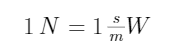that is, the force 1 N  (Newton) on the left side of the equal sign is conventionally regarded as a vector, and the power on the right side is regarded as a scalar. In response to this problem, the author points out that equation (10) must be understood as: if the power on the right-hand side of equation (10) is a scalar, the force 1 N (Newton) on the left-hand side of the equation should be regarded as the strength (scalar) of the force, If the force on the left-hand side of (10) is a vector, then the power 1 W  (watt) on the right-hand side of (10) needs to be treated as a vector that aligns with the direction of the force.

This understanding is in line with the actual situation:

1. Some forces and powers must be described using scalars.

For example, the light emitted by an ideal point light source (such as a very small light bulb, a star seen from a distance) can be measured in watts to measure the total intensity (total power) of its overall instantaneous luminescence, and it can also be measured in Newtons the total light pressure (force). This metered total luminous power and total light pressure have no direction problem and are both scalars.

2) Some forces and powers are more accurate and convenient to use vector descriptions.

For example, if the above-mentioned point light source shoots somewhere outside, a beam flow per unit area that is locally perpendicular to the light rays must have a power (area) density and will generate a local pressure on the area. At this time, the power density and pressure of the beam flow are obviously directional and should be regarded as vectors. If the unit area is a little local part of a sphere surface centered on the light source, then the second type of surface integral can simply be used to prove that the sum of the power densities passing through the sphere surface is equal to the total power of the light source, and it can also be proved that the sum of the pressures passing through the sphere surfaces equal to the total light pressure  of the light source.  When the sphere becomes a smooth closed surface around the light source, the surface integration of the second type above can still be performed, and it can be proved that as long as there are no other light sources or other obstacles in the closed surface, the integral values, namely the total power and total light pressure, remain unchanged. If only a scalar is used to describe the power or light pressure of the local beam, it will bring a lot of troubles and even lead to errors.

3) In many literatures, when referring to force and power in general, the description of directional force and power does not care whether a scalar or a vector is used.

The energy flux (irradiance) of a plane wave is calculated using the Poynting vector, whose magnitude we denote by S. S divided by the speed of light is the density of the linear momentum per unit area (pressure) of the electromagnetic field. So, dimensionally, the Poynting vector is S=power/area=rate of doing work/area=ΔF/ΔtΔx/area, which is the speed of light, c=Δx/Δt, times pressure, ΔF/area. That pressure is experienced as radiation pressure on the surface:where the intensity S (scalar) of the Poyintng vector S is explicitly used to describe the power (area) density of the radiation, while the symbol <S> is used when it is used to represent the pressure, and it is not stated whether this symbol and the corresponding pressure Pincident are a vector or a scalar. So, if Pincident  is considered as a vector, then the radiated power density <S> must be considered as a vector.

For another example, when discussing solar light pressure, Maxwell stated in his important work, <A Treatise on Electricity and Magnetism (1St ed.), Vol. 2>, the beginning of §793 as follows:

Thus, if in strong sunlight the energy of the light which falls on one square foot is 83.4 foot pounds per second, the mean energy in one cubic foot of sunlight is about 0.0000000882 of a foot pound, and the mean pressure on a square foot is 0.0000000882 of a pound weight.

where obviously the radiant power density of sunlight (at the Earth's surface) is expressed using the imperial system, i.e.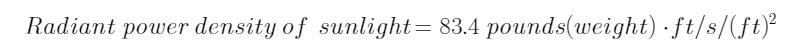The corresponding part in the German translation is

Nimmt man an, dass kraftiges Sunnenlight an einem Quadratmeter in der Secunde 124.1 Kilogrammeter Energie entwickelt, so warden in einem Kubikmeter Sonnenstrahlen 124/30000000 oder 0.00000041 Kilogram Drmeter Energie entachreuck sein, eine zur Fortplanzungstrichtung der Sonnenstrahlen senkrechte Flache pro Quadratmeter erleidet, 0.00000041 Kilogrammeter betragen.

which also is expressed in the more familiar unit system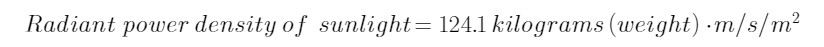The above citation also shows that Maxwell understood the sunlight energy received per cubic meter per second as the light pressure received, which is 0.00000042 kilograms per square meter, seriously underestimating the intensity of the solar light pressure.

At the same time, Bartoli independently used his own unique idea in his work on the same problem to give the power density and pressure of electromagnetic radiation.

Inheriting the ideas of Maxwell and Bartoli, Lebedev directly gave the Maxwell-Bartoli radiation pressure formula in the paper introducing his light pressure experiment(1)

where, according to the custom at the time, V  represents the speed of light.

From Maxwell to Lebedev's expressions, it can be seen that the quantities on both sides of each equation  can be either viewed as both scalars, or viewed as both vectors.

### II. Electromagnetic radiation intensity and light pressure, Einstein's important contribution to the theory of light pressure

As can be seen from the introduction in the previous section, from Maxwell to Lebedev, the light pressure is calculated by dividing the incident power density of the beam by the speed of light. The rationale for this may be the common power expression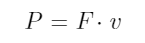（2）

where P (Power) is power. These scholars apparently replaced the velocity v in equation (2) with the speed of light V  to get (1).

The author already pointed out in the first blog post at the beginning of the introduction that if the speed of light V  is used instead of v, it means that the object affected by the beam is the light itself, which is meaningless. Other problems with equation (2) are also pointed out in the third blog post.

Interestingly, the authors found that the term power was hardly seen in the important physics papers in Einstein's corpus, the leading physicist of the 20th century. Nevertheless, his relationship between power and corresponding force is clear. In particular, in his 1905 publication of one of his major works on special relativity, "Zur Elektrodynamik bewegter Körper" (<ON THE ELECTRODYNAMICS OF MOVING BODIES

>), when he demonstrated "the transformation of light energy and the radiation pressure acting on a perfectly reflecting mirror Theory", it is stated on page 915 as follows (in German)In <ON THE ELECTRODYNAMICS OF MOVING BODIES> , the corresponding English content (in page 18-19) is

The energy (measured in the stationary system) which is incident upon unit area of the mirror in unit time is evidently A2(c cos φ−v)/8π. The energy leaving the unit of surface of the mirror in the unit of time is A'''2(−c cos φ''' + v)/8π. The difference of these two expressions is, by the principle of energy, the work done by the pressure of light in the unit of time. If we set down this work as equal to the product Pv, where P is the pressure of light, we obtain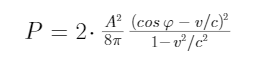In agreement with experiment and with other theories, we obtain to a first approximation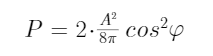In this statement, Einstein referred to "the energy which is incident upon unit area of the mirror in unit time the energy per unit area of the mirror per unit time", "the energy leaving the unit of surface of the mirror in the unit of time", and "the work done by the pressure of light in the unit of time", are obviously the power density.

Einstein put forward the basic principle for the calculation of light pressure in this discussion, that is, "The difference of these two expressions is, by the principle of energy, the work done by the pressure of light in the unit of time. ”

The author points out that in the literature he has seen, unlike previous scholars, Einstein was the first physicist in the world to propose the basic principles of light pressure calculation. It is not difficult to see that the properties of light pressure derived under this principle must be consistent with the results of the Crooks radiometer experiment. Furthermore, the pressure formula derived from this principle should not underestimate light pressure like the Maxwell-Bartoli formula.

Regrettably, the author finds the relevant mathematical derivation unreasonable, because according to this statement, it is impossible to mathematically derive the resulting pressure formula（3）

or（4）

Readers are advised to check this derivation with their own hands-on calculations.

The author believes that the key to the unreasonable mathematical derivation lies in the mathematical expression: "A2(c cos φ - v)/8π " and "A'''2(-c cos φ''' + v)/8π ".

The author doubts whether these two mathematical expressions are appropriate, whether there is a mathematical or physical basis, whether A2(c cos φ - v)2 is miswritten as A2(c cos φ - v), A'''2( -c cos φ''' + v)2 is incorrectly written as A'''2(-c cos φ''' + v), because these mistakes will not only lead to the problem that the energy value may be negative, but also make it impossible to derive the factor (cos φ - v/c)2 in (3) or the factor cos2φ in equation (4).

Another important reason why the two pressure formulas cannot be deduced in this paper is the power density expression Pv which is commonly used in textbooks or literature. This is because there are many special factors in this expression, and it is not an accurate, objective expression of the force-power relationship (see the third blog post listed above).

If the expressions A2(c cos φ - v)/8π and A'''2(-c cos φ''' + v) are respectively replaced by the corresponding power density expressions that are correctly expressed, and the expressions Pv is replaced by the objective equivalence relationship between force and power, then according to the basic principle of light pressure calculation proposed by Einstein, he will definitely derive the pressure formula obtained by the author in the first blog post above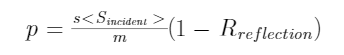(5)

### III. Why can't the word power be found in Landau and Lifshitz's great tome of physics?

Recently, the author noticed with surprise that it is difficult to find a description of the definition and use of power in the mechanics section of existing college physics textbooks. In particular, in the ten-volume masterpiece <Theoretical Physics Course> by the well-known physicist of the former Soviet Union, Lev Davidovich Landau and his student Yevgeny Livschitz, the author could hardly find the term "Power" in mechanics, especially the first volume <Mechanics> .

In reading this work, the authors are deeply aware that it is not the negligence of these great authors, but that they have noticed that with the development of modern physics, the forms and changes of forces and energies (including forces) are becoming more complex, there are also many related phenomena that humans do not know or understand. For example, when it comes to friction, they consider this problem is beyond pure mechanics (see Section 25 in ). The authors speculate that this is one of the reasons why the word "force" is rarely used in this magnum opus, even though it covers a wide range of modern physics.

Because this great tome includes very modern advances in physics, including many areas of Landau's major research results, such as in condensed matter physics , and the extremely modern volume 10 <Physical Kinetics> , although the author does not know much about most of these fields, but is convinced that "power" is a very important physical concept, and that when the theory and application of the equivalence relationship between force and power are further studied, this masterpiece has provided lots of research material.

### IV.  Some inner understandings of human beings on "Power" and "Force"

On the other hand it is not difficult to see that power is indeed covered in detail as an important physical concept in many modern literature and important textbooks. Typical are:

Wikipedia explains the related entry:

https://en.wikipedia.org/wiki/Power_(physics)

and

<Fundamentals of Physics>  by Robert Resnick, David Halliday and Jearl Walker;

<Feynman Lectures on Physics> by Feynman R, Leighton R, and Sands M.

It is interesting and thought-provoking to describe these concepts directly in the language of the heart, if the historical and modern knowledge is synthesized, aside from the definitions expressed through mathematical formulas.

For example，Chinese Wikipedia (https://zh.wikipedia.org/wiki/Power) explains Power as

Power (English: power) is defined as the rate at which energy is converted or used, expressed as the amount of energy per unit time, that is, the rate of work. The SI unit of power is the watt (W), named after the eighteenth-century steam engine designer James Watt. The amount of electric energy converted into heat and light energy by a light bulb in a unit time can be expressed in power. The higher the wattage, the higher the capacity (or electricity) per unit time.

The textual explanation of power in <Fundamentals of Physics> (Chapter 7, p. 166) is

The time rate at which work is done by a force is said to be the power due to the force.

The definition of power on page 13-2 of <Feynman Lectures on Physics> is

F· v is called power: the force acting on an object times the velocIty of the object (vector "dot" product) is the power being delivered to the object by that force. We thus have a marvelous theorem: the rate of change of kinetic energy of an object IS equal to the power expended by the forces acting on it.

It can be clearly seen from all the above textual explanations that in people's minds, power is regarded as a direct manifestation of the action of force, and force is inseparable from the power it consumes or outputs.

After consulting the explanation of Power in Chinese Wikipedia above, as well as https://en.wikipedia.org/wiki/James_Watt, https://en.wikipedia.org/wiki/Horsepower, the author concludes that the physics term "Power" comes from The Scottish engineer, inventor of the improved steam engine, James Watt, and the term was later widely used in mechanical theory to describe aspects such as the output capacity of engines and electricity.

Based on the above information, since the term "Power", a physical concept, first appeared in English, how do people understand this word in the English-speaking world?

According to the authoritative Oxford English Dictionary, the word power is often understood as "strength, power, energy" (see p. 562 of the 7th edition of the dictionary), that is, power is often understood as force. According to the online Google Translate https://translate.google.com/, when "power" is translated into Chinese, the result is often "force". When translated into Russian, it is "сила", which means "force". Likewise, when Google Translate translates the word into other languages, the results mostly contain the meaning of force.

In short, power and force are inseparable.

On the other hand, in the above literature, how to express the understanding of "force" only in words?

The English Wikipedia (https://en.wikipedia.org/wiki/Force) describes "Force" as follows:

In physics, a force is an influence that can change the motion of an object. A force can cause an object with mass to change its velocity (e.g. moving from a state of rest), i.e., to accelerate. Force can also be described intuitively as a push or a pull. A force has both magnitude and direction, making it a vector quantity. It is measured in the SI unit of newton (N). Force is represented by the symbol F (formerly P).

Interestingly, P  is the first letter of the word "Power". Is this pure coincidence?

<Fundamentals of Physics> textual interpretation of "Force" (Chapter 5, p. 94) is

What Is Physics? We have seen that part of physics is a study of motion, including accelerations, which are changes in velocities. Physics is also a study of what can cause an object to accelerate.That cause is a force, which is, loosely speaking, a push or pull on the object. The force is said to act on the object to change its velocity.

Based on the explanations of "force" in the above-mentioned literature, the author has to regret to say that none of these modern explanations mention the relationship between force and power. Analyzing the reasons, these explanations are all influenced by Newton's second law, and the explanations are all overly modern. The author argues that these modern explanations forget about earlier understandings of statics, excluding statics from mechanics, just as Landau and Lifshitz dealt with friction.

There is no doubt that statics is the most classical and important part of mechanics.

In the first blog post mentioned above, the author has demonstrated that the so-called "static force" also does work, and it is not purely "static".

The actual experience of human beings is: whether it is a human being or an inanimate matter, it must have different performances when it is subjected to static force and when it is not subjected to static force, and the longer it is subjected to static force, the more obvious these performances are. This is clear evidence that static force is doing work, but these evidences are often shown in the motion of the microscopic material world and are often ignored by people!

The author is convinced that when this neglect is faced, "static force" will no longer be regarded as an ancient and discardable concept of mechanics, but on the contrary, it should be an important object of modern physics and mechanics research. Therefore, the author emphasizes again that force is equivalent to power.

## nslation results

### V.   Summary

Einstein discovered the equivalence relationship between mass and energy with different dimensions, so isn’t there a similar equivalence relationship between force and power with different dimensions?

Without force there is no power, without power there is no force, force and power describe the same physical object!

If the equivalence relationship between force and power is faced, it is very meaningful for the theoretical and applied research of physics and mechanics. The author has pointed out that this relationship shows that the "static force" is also doing work, which solves the problem of calculating the energy consumption of a person with a load, and also gives a more reasonable radiation pressure formula based on this relationship.

According to this relationship, the output power of the engine can be directly regarded as the output force. In engineering matters, most engines transmit their power or force through the rotors in them and the wheels of the conveyor belt. In the past, when people calculated the "force" transmitted through the conveyor belt, it was necessary to take into account a series of special factors such as the radius of the conveyor wheel, the rotation speed (depending on the resistance encountered), the torque, etc., which undoubtedly led to the misunderstanding that two engines with the same output power could output different "forces".  In fact, the equivalence relationship between force and power can be used to simply, objectively and clearly explain how many watts the output power of the engine is, and how much Newton force the output force only corresponds to, which is the characteristic of the engine itself.

The equivalence relationship between force and power will also be of great significance to the study of the evolution of the universe and the evolution of celestial bodies (including planets, galaxies, black holes, etc.). As a counter-example, the famous physicist, Arthur Eddington, in his famous book <The internal constitution of the stars>, like Maxwell, greatly underestimated the radiation pressure (see Chapter 2 of this work, 27-28 page, §21), which undoubtedly also underestimates the role of radiation pressure in the equilibrium inside.

"Energy" and "power" are obviously not the same physical object, and the "equivalence relationship between mass and energy" and "equivalence relationship between force and power" are obviously not the same physical object. These two types of equivalence relations are of great significance in physics.

Reference:

 J. C. Maxwell, A Treatise on Electricity and Magnetism (1St ed.), Vol. 2, Oxford, 1873.

 J. C.  Maxwell, Lehrbuch der Elektricitiit und des Magnetismus. Deutsch von B. Weinstein, Berlin 1883.

 A. Bartoli, Ezner's Bep. d. Phys. 21. p. 198. 1884, übersetzt aus Nuovo Cimento 16. p. 195. 1883.

  P. Lebedew: Untersuchen uber die druckkrafte des Lichtes, Annalen der Physik 46, 432 (1901).

  Einstein, Albert (1905d) [Manuscript received: 30 June 1905]. Written at Berne, Switzerland. "Zur Elektrodynamik bewegter Körper". Annalen der Physik (Submitted manuscript) (in German). Hoboken, New Jersey (published 10 March 2006). 322 (10): 891–921.

 Landau, Lev D.; Lifshitz, Evgeny M. (1976). Mechanics. Vol. 1 (3rd ed.). Butterworth-Heinemann. ISBN 978-0-7506-2896-9.

 Lifshitz, Evgeny M.; Pitaevskii, Lev P. (1980). Statistical Physics, Part 2: Theory of the Condensed State. Vol. 9 (1st ed.). Butterworth-Heinemann. ISBN 978-0-7506-2636-1.

 Lifshitz, Evgeny M.; Pitaevskii, Lev P. (1981). Physical Kinetics. Vol. 10 (1st ed.). Pergamon Press. ISBN 978-0-7506-2635-4.

  Robert Resnick, David Halliday, Jearl Walker, Fundamentals of Physics, Wiley & Sons, Incorporated, John, (2000), ISBN: 0471332356, ISBN13: 9780471332350

 Feynman R, Leighton R, and Sands M. The Feynman Lectures on Physics. Three volumes 1964, 1966. Library of Congress Catalog Card No. 63-20717, ISBN 0-201-02115-3 (1970 paperback three-volume set)
 <Paperback Oxford English Dictionary>, seventh edition, edited by Aauice Watte, Oxfoord University Press, 2022, ISBN: 978-0-19-964094-2.

 Arthur S. Eddington, The internal constitution of the stars,with a new forwaord by S. Chandrasekhar, Cambridge University Press, Cambridge, New York, New Rochelle, Melbourne, Sydney,1988, ISBN 0 521 33708 9

## 再论力-功率等价关系

Email：keying.guan@gmail.com

二   电磁辐射强度与光压，爱因斯坦对光压理论的重要贡献

三   为何在朗道与栗弗席兹的物理巨著中找不到功率一词？

四   人类对功率与力的一些内在理解

五   总结

1. 没有不做功的力--马德堡半球实验、举重运动消耗的卡路里及辐射压公式 2022-07-01

2. Force is equivalent to Power 2022-07-02

### 一      力与功率是矢量还是标量1）有些力与功率必须使用标量描述。

2）有些力与功率使用矢量描述更准确、更方便。

3）在许多文献中，在泛指力和功率时，对有方向的力与功率的描述并不在意使用标量还是矢量

The energy flux (irradiance) of a plane wave is calculated using the Poynting vector, whose magnitude we denote by S. S divided by the speed of light is the density of the linear momentum per unit area (pressure) of the electromagnetic field. So, dimensionally, the Poynting vector is S=power/area=rate of doing work/area=ΔF/ΔtΔx/area, which is the speed of light, c=Δx/Δt, times pressure, ΔF/area. That pressure is experienced as radiation pressure on the surface:Thus, if in strong sunlight the energy of the light which falls on one square foot is 83.4 foot pounds per second, the mean energy in one cubic foot of sunlight is about 0.0000000882 of a foot pound, and the mean pressure on a square foot is 0.0000000882 of a pound weight.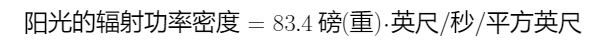Nimmt man an, dass kraftiges Sunnenlight an einem Quadratmeter in der Secunde 124.1 Kilogrammeter Energie entwickelt, so warden in einem Kubikmeter Sonnenstrahlen 124/30000000 oder 0.00000041 Kilogram Drmeter Energie entachreuck sein, eine zur Fortplanzungstrichtung der Sonnenstrahlen senkrechte Flache pro Quadratmeter erleidet, 0.00000041 Kilogrammeter betragen.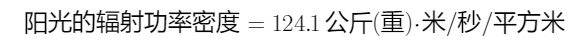（1）

### 二    电磁辐射强度与光压，爱因斯坦对光压理论的重要贡献（2）（3）（4）(5)

### 四     人类对功率与力的一些内在理解

Wikipedia 对相关的词条解释:

## Translation results,

<Fundamentals of Physics> 对功率的文字解释（第七章，166页）为

The time rate at which work is done by a force is said to be the power due to the force.

<Feynman Lectures on Physics> 的第13-2页中对功率的定义是

F· v is called power: the force acting on an object times the velocIty of the object (vector "dot" product) is the power being delivered to the object by that force. We thus have a marvelous theorem: the rate of change of kinetic energy of an object IS equal to the power expended by the forces actmg on it.

F·v 称为功率：作用在物体上的力乘以物体的速度（矢量“点”积）就是该力传递给物体的功率。 因此，我们有一个奇妙的定理：物体的动能变化率等于作用在其上的力所消耗的功率。

In physics, a force is an influence that can change the motion of an object. A force can cause an object with mass to change its velocity (e.g. moving from a state of rest), i.e., to accelerate. Force can also be described intuitively as a push or a pull. A force has both magnitude and direction, making it a vector quantity. It is measured in the SI unit of newton (N). Force is represented by the symbol F (formerly P).

<Fundamentals of Physics> 对“力” (Force) 的文字解释（第五章，94页）为

What Is Physics? We have seen that part of physics is a study of motion, including accelerations, which are changes in velocities. Physics is also a study of what can cause an object to accelerate.That cause is a force, which is, loosely speaking, a push or pull on the object. The force is said to act on the object to change its velocity.

### 五  总结

“能量” 与 “功率” 显然不是相同的物理对象，“质量与能量之间的等价关系” 和 “力与功率的等价关系” 也显然不是同样的物理对象。这两类等价关系在物理学中有着极重要的意义。

 J. C. Maxwell, A Treatise on Electricity and Magnetism (1St ed.), Vol. 2, Oxford, 1873.

 J. C.  Maxwell, Lehrbuch der Elektricitiit und des Magnetismus. Deutsch von B. Weinstein, Berlin 1883.

 A. Bartoli, Ezner's Bep. d. Phys. 21. p. 198. 1884, übersetzt aus Nuovo Cimento 16. p. 195. 1883.

  P. Lebedew: Untersuchen uber die druckkrafte des Lichtes, Annalen der Physik 46, 432 (1901).

  Einstein, Albert (1905d) [Manuscript received: 30 June 1905]. Written at Berne, Switzerland. "Zur Elektrodynamik bewegter Körper"Annalen der Physik (Submitted manuscript) (in German). Hoboken, New Jersey (published 10 March 2006). 322 (10): 891–921.

 范岱年 赵中立 许良英编译，爱因斯坦文集，第二卷，商务印数馆出版，1977年第一版，统一书号：2017●184

 Landau, Lev D.; Lifshitz, Evgeny M. (1976). Mechanics. Vol. 1 (3rd ed.). Butterworth-Heinemann. ISBN 978-0-7506-2896-9.

 Lifshitz, Evgeny M.; Pitaevskii, Lev P. (1980). Statistical Physics, Part 2: Theory of the Condensed State. Vol. 9 (1st ed.). Butterworth-Heinemann. ISBN 978-0-7506-2636-1.

 Lifshitz, Evgeny M.; Pitaevskii, Lev P. (1981). Physical Kinetics. Vol. 10 (1st ed.). Pergamon Press. ISBN 978-0-7506-2635-4.

  Robert Resnick, David Halliday, Jearl Walker, Fundamentals of Physics, Wiley & Sons, Incorporated, John, (2000), ISBN: 0471332356, ISBN13: 9780471332350  Feynman R, Leighton R, and Sands M. The Feynman Lectures on Physics. Three volumes 1964, 1966. Library of Congress Catalog Card No. 63-20717, ISBN 0-201-02115-3 (1970 paperback three-volume set)
 <Paperback Oxford English Dictionary>, seventh edition, edited by Aauice Watte, Oxfoord University Press, 2022, ISBN: 978-0-19-964094-2.

 Arthur S. Eddington, The internal constitution of the stars,with a new forwaord by S. Chandrasekhar, Cambridge University Press, Cambridge, New York, New Rochelle, Melbourne, Sydney,1988, ISBN 0 521 33708 9

https://blog.sciencenet.cn/blog-553379-1346635.html

## 全部精选博文导读

GMT+8, 2023-11-29 09:23